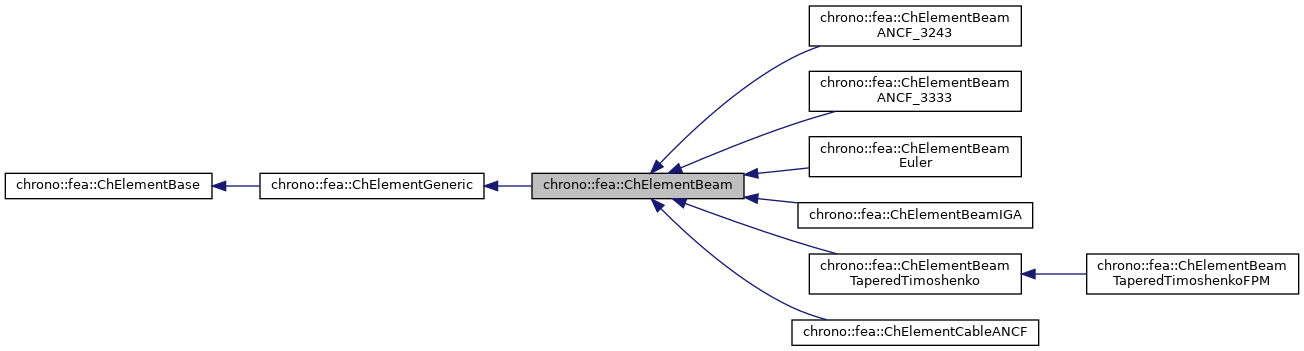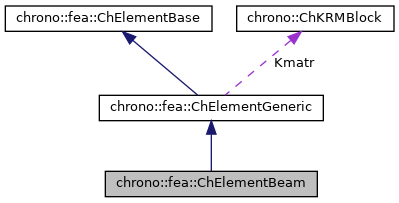chrono::fea::ChElementBeam Class Referenceabstract

## Description

Base class for most structural elements of 'beam' type.

#include <ChElementBeam.h>

Inheritance diagram for chrono::fea::ChElementBeam:[legend]
Collaboration diagram for chrono::fea::ChElementBeam:[legend]

## Public Member Functions

virtual void EvaluateSectionDisplacement (const double eta, ChVector<> &u_displ, ChVector<> &u_rotaz)=0
Gets the xyz displacement of a point on the beam line, and the rotation RxRyRz of section plane, at abscissa 'eta'. More...

virtual void EvaluateSectionFrame (const double eta, ChVector<> &point, ChQuaternion<> &rot)=0
Gets the absolute xyz position of a point on the beam line, and the absolute rotation of section plane, at abscissa 'eta'. More...

virtual void EvaluateSectionForceTorque (const double eta, ChVector<> &Fforce, ChVector<> &Mtorque)=0
Gets the force (traction x, shear y, shear z) and the torque (torsion on x, bending on y, on bending on z) at a section along the beam line, at abscissa 'eta'. More...

virtual void EvaluateSectionStrain (const double eta, ChVector<> &StrainV)=0
Gets the axial and bending strain of the ANCF "cable" element.

double GetMass ()
The full mass of the beam, (with const. section, density, etc.)

double GetRestLength ()
The rest length of the bar.

void SetRestLength (double ml)
Set the rest length of the bar (usually this should be automatically done when SetupInitial is called on beams element, given the current state, but one might need to override this, ex for precompressed beams etc).Public Member Functions inherited from chrono::fea::ChElementGeneric
ChKblockGenericKstiffness ()
Access the proxy to stiffness, for sparse solver.

virtual void EleIntLoadResidual_F (ChVectorDynamic<> &R, const double c) override
Add the internal forces (pasted at global nodes offsets) into a global vector R, multiplied by a scaling factor c, as R += forces * c This default implementation is SLIGHTLY INEFFICIENT.

virtual void EleIntLoadResidual_Mv (ChVectorDynamic<> &R, const ChVectorDynamic<> &w, const double c) override
Add the product of element mass M by a vector w (pasted at global nodes offsets) into a global vector R, multiplied by a scaling factor c, as R += M * w * c This default implementation is VERY INEFFICIENT.

virtual void EleIntLoadResidual_F_gravity (ChVectorDynamic<> &R, const ChVector<> &G_acc, const double c) override
Add the contribution of gravity loads, multiplied by a scaling factor c, as: R += M * g * c This default implementation is VERY INEFFICIENT. More...

virtual void ComputeGravityForces (ChVectorDynamic<> &Fg, const ChVector<> &G_acc) override
Compute the gravitational forces. More...

virtual void ComputeMmatrixGlobal (ChMatrixRef M) override
Calculate the mass matrix, expressed in global reference. More...

virtual void InjectKRMmatrices (ChSystemDescriptor &descriptor) override
Tell to a system descriptor that there are item(s) of type ChKblock in this object (for further passing it to a solver)

virtual void KRMmatricesLoad (double Kfactor, double Rfactor, double Mfactor) override
Add the current stiffness K and damping R and mass M matrices in encapsulated ChKblock item(s), if any. More...

virtual void VariablesFbLoadInternalForces (double factor=1.) override
Add the internal forces, expressed as nodal forces, into the encapsulated ChVariables.

virtual void VariablesFbIncrementMq () override
Add M*q (internal masses multiplied current 'qb').Public Member Functions inherited from chrono::fea::ChElementBase
virtual int GetNnodes ()=0
Get the number of nodes used by this element.

virtual int GetNdofs ()=0
Get the number of coordinates in the field used by the referenced nodes. More...

virtual int GetNdofs_active ()
Get the actual number of active degrees of freedom. More...

virtual int GetNodeNdofs (int n)=0
Get the number of coordinates from the specified node that are used by this element. More...

virtual int GetNodeNdofs_active (int n)
Get the actual number of active coordinates from the specified node that are used by this element. More...

virtual std::shared_ptr< ChNodeFEAbaseGetNodeN (int n)=0
Access the nth node.

virtual void GetStateBlock (ChVectorDynamic<> &mD)=0
Fill the D vector with the current field values at the nodes of the element, with proper ordering. More...

virtual void ComputeNodalMass ()
Compute element's nodal masses.

virtual void ComputeKRMmatricesGlobal (ChMatrixRef H, double Kfactor, double Rfactor=0, double Mfactor=0)=0
Set H as the stiffness matrix K, scaled by Kfactor. More...

virtual void ComputeInternalForces (ChVectorDynamic<> &Fi)=0
Compute the internal forces. More...

virtual void Update ()
Update, called at least at each time step. More...

virtual void EleDoIntegration ()
This is optionally implemented if there is some internal state that requires integration.

## Protected Attributes

double mass

double lengthProtected Attributes inherited from chrono::fea::ChElementGeneric
ChKblockGeneric Kmatr

## ◆ EvaluateSectionDisplacement()

 virtual void chrono::fea::ChElementBeam::EvaluateSectionDisplacement ( const double eta, ChVector<> & u_displ, ChVector<> & u_rotaz )
pure virtual

Gets the xyz displacement of a point on the beam line, and the rotation RxRyRz of section plane, at abscissa 'eta'.

Note, eta=-1 at node1, eta=+1 at node2.

## ◆ EvaluateSectionForceTorque()

 virtual void chrono::fea::ChElementBeam::EvaluateSectionForceTorque ( const double eta, ChVector<> & Fforce, ChVector<> & Mtorque )
pure virtual

Gets the force (traction x, shear y, shear z) and the torque (torsion on x, bending on y, on bending on z) at a section along the beam line, at abscissa 'eta'.

Note, eta=-1 at node1, eta=+1 at node2. Results are not corotated, and are expressed in the reference system of beam.

## ◆ EvaluateSectionFrame()

 virtual void chrono::fea::ChElementBeam::EvaluateSectionFrame ( const double eta, ChVector<> & point, ChQuaternion<> & rot )
pure virtual

Gets the absolute xyz position of a point on the beam line, and the absolute rotation of section plane, at abscissa 'eta'.

Note, eta=-1 at node1, eta=+1 at node2.

The documentation for this class was generated from the following file:
• /builds/uwsbel/chrono/src/chrono/fea/ChElementBeam.h